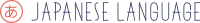0

## Please login or register to vote for this query.

(click on this box to dismiss)Q&A for students, teachers, and linguists wanting to discuss the finer points of the Japanese language

``````With
members as (
select u.Id as Id,
min(p.CreationDate) as FirstPostDate
from Users u join PostsWithDeleted p
on u.id = p.OwnerUserId
where (DATEDIFF(hh, '2012/01/01 00:00', u.CreationDate) > 0)
group by u.Id
),
posttimes as (
select m.Id,
p.Score,
DATEDIFF(hh, m.FirstPostDate, p.CreationDate) as timediff,
case
when m.FirstPostDate = p.CreationDate
then 1
else 0
end as firstpost
from members m join PostsWithDeleted p
on m.Id = p.OwnerUserId
where p.PostTypeId = 2
),
sumtimes as (
select Id,
sum(case when timediff =0 then 1 else 0 end) firstHourPosts,
sum(case when timediff <=23 then 1 else 0 end) firstDayPosts,
sum(case when timediff <=167 then 1 else 0 end) firstWeekPosts
from posttimes
group by Id
),
userdistribution as (
select s.firstDayPosts,
count(u.Id)       as nTot,
sum(case when u.Reputation < 50 then 1 else 0 end) as nLow,
sum(case when u.Reputation > 1000 then 1 else 0 end) as nHigh
from sumtimes s join users u
on s.Id = u.Id
group by s.firstDayPosts
),
postdistribution as (
select timediff - firstpost as timediff,
count(Id)       as nTot,
sum(case when Score < 1 then 1 else 0 end) as nLow,
sum(case when Score > 5 then 1 else 0 end) as nHigh
from posttimes
group by timediff - firstpost
)

select
firstDayPosts,
(1-1.00*( sum(nTot) over (order by firstDayPosts) )/(select sum(nTot) from userdistribution)) as fTot,
(1-1.00*( sum(nLow) over (order by firstDayPosts) )/(select sum(nLow) from userdistribution)) as fLow,
(1-1.00*( sum(nHigh) over (order by firstDayPosts) )/(select sum(nHigh) from userdistribution)) as fHigh
from userdistribution
order by firstDayPosts``````

### Enter Parameters

Options:
-Hold tight while we fetch your results
:records returned in :time ms:cached